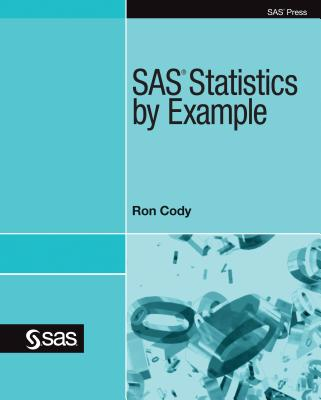### SAS Statistics by Example### Huge savings for students

Each student receives a 50% discount off of most books in the HSG Book Store. During class, please ask the instructor about purchase details.
 List Price: \$49.95 Price: \$24.98 You Save: \$24.98
6In SAS Statistics by Example, Ron Cody offers up a cookbook approach for doing statistics with SAS. Structured specifically around the most commonly used statistical tasks or techniques--for example, comparing two means, ANOVA, and regression--this book provides an easy-to-follow, how-to approach to statistical analysis not found in other books.

For each statistical task, Cody includes heavily annotated examples using ODS Statistical Graphics procedures such as SGPLOT, SGSCATTER, and SGPANEL that show how SAS can produce the required statistics. Also, you will learn how to test the assumptions for all relevant statistical tests. Major topics featured are correlation, inferential statistics, descriptive statistics, categorical data analysis, simple linear regression, comparing means, multiple regression, logistic regression, non-parametric tests, and power and sample size.

This is not a book that teaches statistics. Rather, SAS Statistics by Example is perfect for intermediate to advanced statistical programmers who know their statistics and want to use SAS to do their analyses.

SAS Institute